# Brushed DC Motor Theory

## Introduction

The specific type of motor we are addressing is the permanent magnet brushed DC motor (PMDC). These motors have two terminals. Applying a voltage across the terminals results in a proportional speed of the output shaft.

There are two pieces to the motor: 1) stator and 2) rotor. The stator includes the housing, windings and brushes. The rotor consists of the output shaft, windings and commutators. The image below shows a cut-away view of a Maxon motor. Note this picture has a gearbox and encoder attached to the motor.

## Motor Physics

The forces inside a motor that cause the rotor to rotate are called Lorentz Forces. If an electron is moving through an electric field, it experiences a force that is perpendicular to both the magnetic field and the direction it's moving. If we have a wire instead of a single electron, the wire experiences a force equal to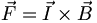$\vec F = \vec I \times \vec B\,$

You can easily find the direction of this force using the Right Hand Rule. The Right Hand Rule states that if you point your right hand fingers along the direction of current, I, and curl them towards the direction of the magnetic flux, B, the direction of force is along the thumb. See the picture below.

Now, imagine this single wire is replaced with a loop of wire. Between the magnets poles, this looks like two wires with current flowing in opposite directions. The forces on the wires cause the loop to rotate with forces as shown.

This coil is attached to the rotor and rotates. As it does so, the magnitude and direction of the force on the wires remains constant. However, the resultant torque varies with the angle. Look at the picture below. When the coil starts, there is maximum torque. As the coil moves, the moment arm is reduced and the torque decreases. Finally, when the coil is vertical (in the picture), there is no torque.

To keep a (almost) constant torque on the rotor, there are two things that happen. First, the current through the coil is reversed every half turn. So instead of an alternating torque like the one in the first figure below, the torque is always in the same direction. Also, additional coils can be used. When these coils are offset at different angles around the motor, the resultant torque becomes the sum of the colored torque curves in the figure below. The resultant torque is alway greater than zero, but is not constant. This variation is called torque ripple.

The process of switching current direction is called commutation. To switch the direction of curent, brushed DC motors use brushes and commutators. Commutation can also be done electronically (see Brushless DC Motors). The following diagram shows how brushes and commutators work.

### Equations

We start by writing the equation for conservation of energy in the motor. The power is input as electrical power,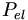$P_{el}\,$ and the motor converts that to mechanical power,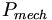$P_{mech}\,$. However, some of the power is lost as heat,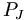$P_J\,$.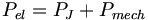$P_{el}=P_J+P_{mech}\,$

We can re-write this in terms of electrical and mechanical quantities as$iv=i^2R+\tau\omega\,$

The wire coils have both a resistance, R, and an inductance, L. When the motor is turning, the current is switching, causing a voltage,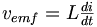$\begin{matrix}v_{emf} = L \frac{di}{dt}\end{matrix}$

This voltage is called the back-emf (electromotive force). The motor constants relate various quantities in a motor. They are a property of the design and material of each individual motor. The speed constant relates the back-emf to the output speed of the shaft,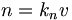$n=k_nv\,$

where n is the rotation in revolutions-per-minute (rpm). The torque constant relates the input current (in the windings) to the torque of the output,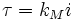$\tau = k_Mi\,$

These two constants are actually the same number with different units. The relationship between the two is

k_n \cdot k_M = \begin{matrix} \frac{30,000}{\pi} \end{matrix}

This voltage,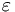$\varepsilon$, is working against the voltage we apply across the terminals, and so,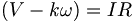$\begin{matrix}(V- k\omega) = IR\end{matrix}$,

where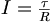$\begin{matrix}I = \frac{\tau}{R}\end{matrix}$

which implies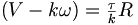$\begin{matrix}(V-k\omega) = \frac{\tau}{k}R\end{matrix}$.

The maximum or stall torque is the torque at which ω = 0 or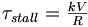$\begin{matrix}\tau_{stall} = \frac{kV}{R}\end{matrix}$,

and the stall or starting current,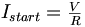$\begin{matrix}I_{start} = \frac{V}{R}\end{matrix}$$\begin{matrix}\omega_{no-load} = \frac{V}{k}\end{matrix}$,

is the maximum speed the motor can run. Given a constant voltage, the motor will settle at a constant speed, just like a terminal velocity. If we plot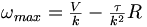$\begin{matrix}\omega_{max} = \frac{V}{k} - \frac{\tau}{k^2}R\end{matrix}$,

we can get a linear speed-torque curve. This line is the dashed line shown in the dc motor speed-torque graph for a Maxon brushed DC motor shown below:

### Units

Here are the different units for the torque, current and voltage

Parameter Symbol Common Units
Torque τ Nm (=kgm/s^2*m), kgfm(=9.8 times Nm), gfcm, mNm, etc
Current I Amperes(Amps), mA
Voltage V Volts
Mechanical Power τω 1 Nm/sec = 1 watt
Electrical Power VI 1 volt*amp = 1 watt